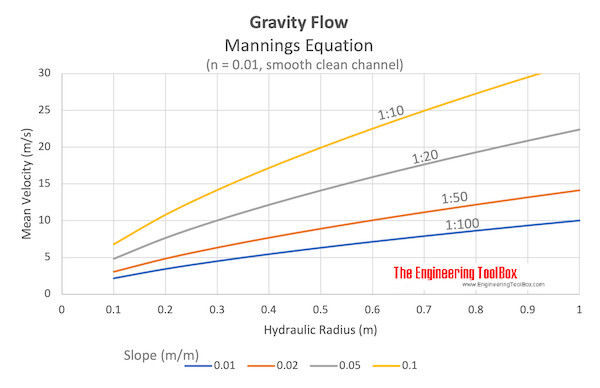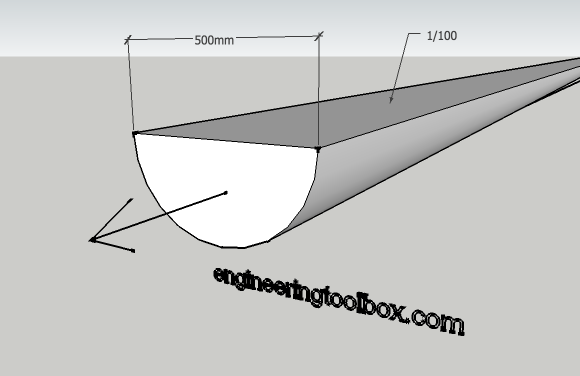Engineering ToolBox - Resources, Tools and Basic Information for Engineering and Design of Technical Applications!

# Manning's Formula and Gravity Flow

## Calculate cross-sectional average velocity flow in open channels.

Manning's equation is an empirical equation that can be used to calculate cross-sectional average velocity flow in open channels

v = (kn / n) Rh2/3 S1/2                       (1)

where

v = cross-sectional mean velocity (ft/s, m/s)

kn = 1.486 for English units and kn = 1.0 for SI units

n = Manning coefficient of roughness - ranging from 0.01 (a clean and smooth channel) to 0.06 (a channel with stones and debris, 1/3 of vegetation)

Rh = hydraulic radius (ft, m)

S = slope - or gradient - of pipe (ft/ft, m/m)

The Manning coefficients n in the English (Imperial) are equal to the coefficients in the SI system. The Manning coefficient is not dimensionless but the units are often omitted.

Units for (kn / n) in the SI system vs. the Imperial system can be expressed as:

(kn / n) = m1/3 / s = 1.4859 ft1/3 / s

Hydraulic radius can be expressed as

Rh = A / Pw                             (2)

where

A = cross sectional area of flow (ft2, m)

Pw = wetted perimeter (ft, m)The volume flow in the channel can be calculated as

q = A v = A (kn / n) Rh2/3 S1/2                         (3)

where

q = volume flow (ft3/s, m3/s)

A = cross-sectional area of flow (ft2, m2)

### Example - Flow in an Open Channel

A channel with the shape of an half circle is 100% filled. The diameter of the half circle is 500 mm (0.5 m) and the channel is made of concrete with Manning coefficient 0.012. The slope of the channel is 1/100 m/m.make 3D models with the free Engineering ToolBox Sketchup Extension!

The cross section area of the half circle flow can be calculated as

A = (0.5 π ((0.5 m) / 2)2)

= 0.098 m2

The wetted perimeter of the half circle flow can be calculated as

P = 0.5 2 π (0.5 m) / 2)

= 0.785 m

The hydraulic radius of the channel can be calculated from (2) as

Rh = A / P

= (0.098 m2) / (0.785 m)

= 0.125 m

The cross sectional mean velocity can be calculated from (1) as

v = (kn / n) Rh2/3 S1/2

= (1.0 / 0.012) (0.125 m)2/3 (1/100 m/m)1/2

= 2.1 m/s

The volume flow can be calculated from (3) as

q = A v

=  (0.098 m2) (2.1 m/s)

= 0.20 m3/s

### Gravity Flow Calculator - Half Filled Circular Pipe

The Gravity Flow Calculator is based on the equations and the example above above. It's valid for half filled circular pipe.

Diameter of pipe (m, ft)

Slope (m/m, ft/ft)

n (1.0 for SI units, 1.486 for Imperial units)

Manning coefficient of roughness

## Related Topics

• ### Flow Measurement

Flow metering principles - Orifice, Venturi, Flow Nozzles, Pitot Tubes, Target, Variable Area, Positive Displacement, Turbine, Vortex, Electromagnetic, Ultrasonic Doppler, Ultrasonic Time-of-travel, Mass Coriolis, Mass Thermal, Weir V-notch, Flume Parshall and Sluice Gate flow meters and more.
• ### Fluid Mechanics

The study of fluids - liquids and gases. Involving velocity, pressure, density and temperature as functions of space and time.

## Related Documents

• ### California Pipe Flow Metering Method

Calculate the discharge length from the open end of a partially filled horizontal pipe.
• ### Chezys Conduit Flow Equation

Calculating volume flow and velcity in open conduits.
• ### Flow Section Channels - Geometric Relationships

Geometric relationships like area, wetted perimeter and hydraulic diameter.
• ### Froude Number

Introduction to the Froude Number.
• ### Hazen-Williams Friction Loss Equation - calculating Head Loss in Water Pipes

Friction head loss (ftH2O per 100 ft pipe) in water pipes can be estimated with the empirical Hazen-Williams equation.
• ### Manning's Roughness Coefficients

Manning's roughness coefficients for some common materials.
• ### Pipe Equations

Calculate cross-sectional areas, weight of empty pipes, weight of pipes filled with water, inside and outside surface areas.
• ### Rainfall on Roofs and Gutter Slopes

Draining of roof areas and recommended gutter slopes
• ### Sewage Sludge Viscosity

Viscosity of sewage sludge vs. content of solids.
• ### Sewer Pipes - Capacities vs. Slope

Carrying capacities of sewer and wastewater pipes - gpm and liter per second.
• ### Storm-Water Conductors

Roof drain capacities.
• ### Storm-Water Runoff Coefficients

Storm-water runoff coefficients for various surfaces
• ### Surface Roughness vs. Mechanical Processing

Mechanical production methods and their surface roughness.
• ### Volume Flow - Online Unit Converter

Convert between volume flow units like gpm, liter/sec, cfm, m3/h.

## Engineering ToolBox - SketchUp Extension - Online 3D modeling!

Add standard and customized parametric components - like flange beams, lumbers, piping, stairs and more - to your Sketchup model with the Engineering ToolBox - SketchUp Extension - enabled for use with older versions of the amazing SketchUp Make and the newer "up to date" SketchUp Pro . Add the Engineering ToolBox extension to your SketchUp Make/Pro from the Extension Warehouse !

We don't collect information from our users. More about

## Citation

• The Engineering ToolBox (2004). Manning's Formula and Gravity Flow. [online] Available at: https://www.engineeringtoolbox.com/mannings-formula-gravity-flow-d_800.html [Accessed Day Month Year].

Modify the access date according your visit.

9.19.12